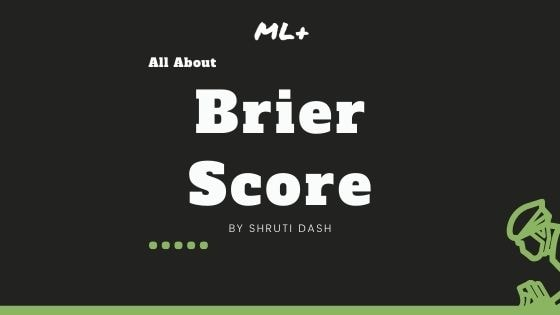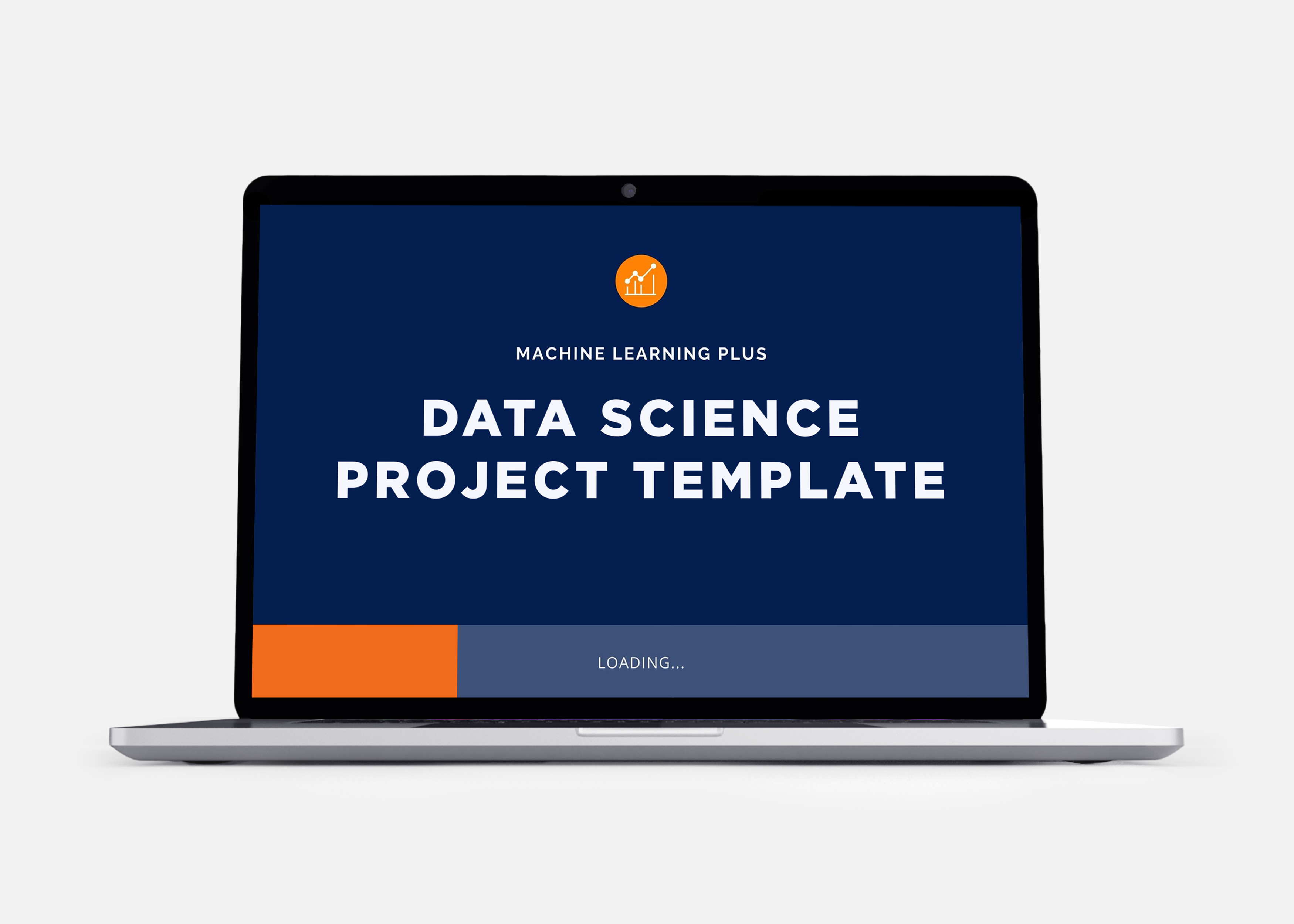# Brier Score – How to measure accuracy of probablistic predictionsBrier score is an evaluation metric that is used to check the goodness of a predicted probability score. This is very similar to the mean squared error, but only applied for prediction probability scores, whose values range between 0 and 1.## Overview

In this tutorial, you will understand:

1. What is Brier score?
2. How is Brier score calculated?
3. Implementation using scikit-learn’s in-built function
4. Brier Skill Score

Get FREE 30 DAY ACCESS  to Structured learning path for ML in Python (Beginner to Pro) –  Start as an absolute beginner and graduate over 15 courses to solve Industrial Projects like a real Data Scientist. Includes query support over chat.

## 1. What is Brier Score?

Brier score is a type of evaluation metric for classification tasks, where you predict outcomes such as win/lose, spam/ham, click/no-click etc.

It is similar in spirit to the log-loss evaluation metric, but the only difference is that it is gentler than log loss in penalizing inaccurate predictions.

So, what exactly is the formula for Brier score?where, f_t is the predicted value and o_t is observed. N is the number of observations. Brier score calculates the mean squared error between predicted probabilities and the observed values (actuals).

The value of the Brier score is always between 0.0 and 1.0, where a model with perfect skill has a score of 0.0 and the worst has a score of 1.0.

However, it’s hard to tell whether the model is good or bad if the Brier score hovers around the 0.5 mark. In such situations, the “Brier Skill Score” might be useful. Discussed in the last section.

• The skill of a model can be described as the average Brier score across all probabilities predicted for a test dataset.

• The lower the brier score is, the better is the performance or skill of the model.

Example:

1. Best Scenario: If the predicted probability is 1.0 and the user actually clicks (actual = 1), then the Brier Score is 0.0.

2. Worst Scenario: If the predicted probability is 1.0 and the user does not click, then the Brier Score is 1.0.

3. Another Scenario: If the predicted probability is .3 and the user does click, then Brier Score is (.3 – 1.0)^2 = 0.49.

Let’s see how Brier Score is calculated.

## 2. Calculate brier score – Example

According to the definition, you already know that the calculation involves subtracting predicted probability from the original outcome, then squaring it, and finding the mean for all observations in the dataset.

Let’s see how this will look in code. We will define a random array of original outcomes y and an array of predicted probabilities y_preds.

import numpy as np
y = np.array([1, 0, 1, 1, 1, 0, 0, 1, 1, 1])
y_preds = np.array([0.31, 0.22, 0.83, 0.74, 0.91, 0.23, 0.56, 0.76, 0.73, 0.97])
losses = np.subtract(y, y_preds)**2
brier_score = losses.sum()/10
brier_score, losses
#> (0.11269999999999998,
#> array([0.4761, 0.0484, 0.0289, 0.0676, 0.0081, 0.0529, 0.3136, 0.0576,
#> 0.0729, 0.0009]))


We get a brier score of 0.112.

From this score, we can infer that our model has good performance or skill. But this was still done on random arrays.

So then, How to calculate the score for an actual classification problem? There is an in-built function from scikit-learn, brier_score_loss().

## 3. Implementation using sckit-learn’s in-built function

To implement the scikit-learn function we will use a more complex classification dataset called ‘admissions.csv’. This dataset has details of different students’ GRE and TOEFL scores, their university ratings, and scores for their SOP, LOR and CGPA.

We are determining the chances of them having done research during their study.

First, we will import all relevant libraries and the dataset.

import pandas as pd
import matplotlib.pyplot as plt
import seaborn as sns
from sklearn.linear_model import LogisticRegression
from sklearn.model_selection import train_test_split
from sklearn.metrics import brier_score_loss
%matplotlib inline


Import dataset

url = 'https://raw.githubusercontent.com/selva86/datasets/master/Admission.csv'
df.set_index('Serial No.', drop=True, inplace=True)
df = df.drop('Chance of Admit ', axis=1)
dfAs you can see, we have 400 entries in the dataset and the ‘Research’ column are binary.

Let’s divide the dataset into train and test sets and calculate the brier score using brier_score_loss function from sklearn library.
The brier_score_loss() function takes the probabilities for the positive class only and returns an average score.

X = df.drop("Research", axis=1)

y = df["Research"]


Create training and test sets

np.random.seed(42)

# Split into train & test set
X_train, X_test, y_train, y_test = train_test_split(X, y, test_size=0.2)


Next, fit the data to the Logistic Regression model.

lr = LogisticRegression()
lr.fit(X_train, y_train)
#> LogisticRegression(C=1.0, class_weight=None, dual=False, fit_intercept=True,
#> intercept_scaling=1, l1_ratio=None, max_iter=100,
#> multi_class='warn', n_jobs=None, penalty='l2',
#> random_state=None, solver='warn', tol=0.0001, verbose=0,
#> warm_start=False)


Predict probability score

lr.score(X_test, y_test)
#> 0.725


The accuracy of our model without any tuning is 72.5%. But our aim is to find the brier score loss, so we will first calculate the probabilities for each data entry in X using the predict_proba() function.

probs = lr.predict_proba(X_test)
probs = probs[:, 1] # Keeping only the values in positive label


Then, compute the Brier Score.

loss = brier_score_loss(y_test, probs)
loss
#> 0.18828291612850948


The brier score loss for the above model is 18.8%.

## 4. Brier Skill Score

While the Brier Score (BS) tells you how good a model is, it is still not a relative metric. That is, it does not tell you how good a model is compared to others. A useful metric to compare the performance of one more in comparison with another is the ‘Brier Skill Score’.

Brier Skill Score = 1 – (BS/BS_baseModelPerformance)

A negative value would mean a poorer model than the base. 0 means they both are equal, whereas, positive means, the new model is better than the base model.

Get FREE 30 DAY ACCESS  to Structured learning path for ML in Python (Beginner to Pro) –  Start as an absolute beginner and graduate over 15 courses to solve Industrial Projects like a real Data Scientist. Includes query support over chat.Reference project template for all your Data Science projects. Learn  how to load the data, get an overview of the data. Perform EDA, prepare data, build models & improve model performance.

## Similar Articles

### Logistic Regression – A Complete Tutorial With Examples in RReference project template for all your Data Science projects. Learn  how to load the data, get an overview of the data. Perform EDA, prepare data, build models & improve model performance.Course Preview

## Machine Learning A-Z™: Hands-On Python & R In Data Science

### Free Sample Videos:#### Machine Learning A-Z™: Hands-On Python & R In Data Science#### Machine Learning A-Z™: Hands-On Python & R In Data Science#### Machine Learning A-Z™: Hands-On Python & R In Data Science#### Machine Learning A-Z™: Hands-On Python & R In Data Science